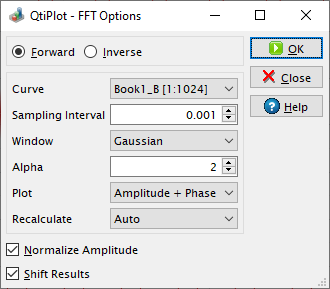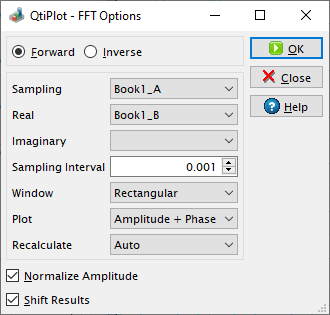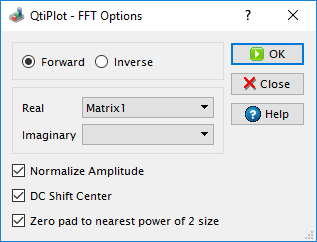# Fast Fourier Transform Dialog

The FFT... dialog box can be used on a plot, on a table or on a matrix. It is used to compute either the direct or inverse FFT. See the FFT section in the Analysis chapter for an example.

Figure 5-78. The FFT... dialog box for a curve.QtiPlot will create a new plot window containing the FFT amplitude curve, along with a hidden table which contains the real part (Re), the imaginary part (Im), the magnitude M = sqrt(Re2 + Im2), the amplitude, and the phase Ph = atan(Im/Re) of the FFT.

If the Normalize Amplitude box is checked, the amplitude curve is normalized to 1. If not, the amplitude is calculated from the magnitude values using the following formulas: Ai = Mi/N for i = 0 or i = N/2 and Ai = 2Mi/N otherwise, where N is the number of samples (data points).

If the Shift Results check box is on, the frequencies are shifted in order to obtain a centered x-scale.

Figure 5-79. The FFT... dialog box for a table.When performing an FFT on a table, you must select the sampling column (X-values) and one (for simple real values) or two (for complex values) columns of Y-values. Complex numbers have the real part of the Y-values in the first column and the imaginary part of the Y-values in the second column. If Y-values are simple reals, you don't have to select a column for the imaginary part. You can leave the combo box for the second column empty in this case.

By default, the Sampling Interval corresponds to the interval between X-values. Giving a smaller value makes no sense, but you can increase this value in order to sample fewer values.

On matrices QtiPlot performs a two dimensional FFT using two sampling intervals: one that corresponds to the interval between the X-values and a second one corresponding to the interval between the Y-values. For more details about the two dimensional FFT please read the following article by Paul Bourke: http://paulbourke.net/miscellaneous/dft/.

Figure 5-80. The FFT... dialog box for a matrix.If the dimensions of the input matrix are not powers of 2, you have the option to resize it for a more efficient FFT, by checking the box Zero-pad to nearest power of 2 size. The new dimensions are calculated to be the closest powers of 2 from the initial dimensions of the matrix and the extra data values used for the FFT are set to zero.

As the result of the FFT operation on a matrix QtiPlot creates a new matrix window containing the amplitude of the FFT, along with two other matrices which contain the real and the imaginary parts of the FFT.